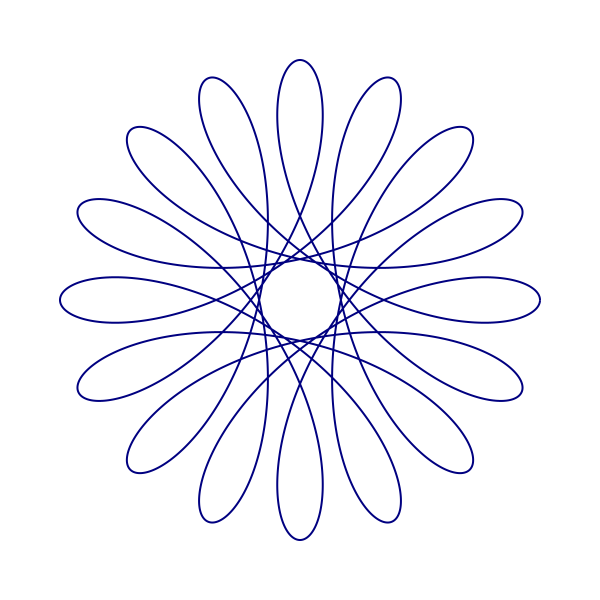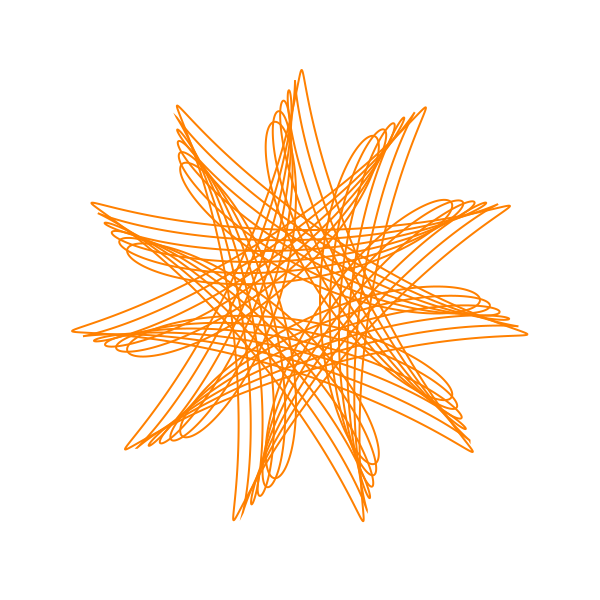# Spirographs

Martin McBride, 2018-04-24
Tags spirograph function plot translation rotation scaling
Categories graphics projects pycairoIn this project you will create code to draw Spirograph images like the one below. You will learn about the Pycairo graphics library, as well as practising some Python coding.

A Spirograph is a toy that uses plastic cogs and coloured pens to create complex patterns. Using Python code to do this is even better, you can create more intricate patterns with more colours and detail.

## Setting up the canvas

Here we will set up our Pycairo canvas, in a similar way to the Pycairo tutorial (it might be a good idea to take a look through the tutorial before attempting this project).

First we set up the canvas size and resolution, and create the canvas and context:

import cairo
import math

WIDTH = 30
HEIGHT = 30
PIXEL_SCALE = 20

surface = cairo.ImageSurface(cairo.FORMAT_RGB24,
WIDTH*PIXEL_SCALE,
HEIGHT*PIXEL_SCALE)
ctx = cairo.Context(surface)
ctx.scale(PIXEL_SCALE, PIXEL_SCALE)


We set the width and height of the image to 30 "units", and set each unit (the pixel scale) to 20, so our image isn 600 (30*20) pixels square. The scaling function means that we can work in units rather than pixels. This makes it easier if we want to make a higher resolution image later, for example if we change the pixel scale to 100 we will get the same image scaled up to 3000 pixels square.

Next we paint a white rectangle the size of the canvas, to give us a white background. We also translate the canvas so that the point (0, 0) is in the centre of the canvas:

ctx.set_source_rgb(1, 1, 1)        # White
ctx.rectangle(0, 0, WIDTH, HEIGHT)
ctx.fill()

ctx.translate(WIDTH/2, HEIGHT/2)


## Creating the Spirograph shape

A spirograph is described by the following parametric equations:

x(t) = (a - b) * math.cos(t) + d * math.cos((a - b)/b * t)
y(t) = (a - b) * math.sin(t) - d * math.sin((a - b)/b * t)


a, b and c are constants that control the shape. t is the equation parameter - a good way to think of it as being time. As time progresses (as t increases), both x and y change, and the point (x, y) moves around the canvas creating a shape.

In fact, computers can't really draw curves. What we will actually do is calculate lots of points on the curve, very close together, and join them together with straight lines. This gives the illusion of a curve.

## Calculating the points

The function create_spiro calculate the points and returns a list if (x, y) tuples. We loop over all values of t from 0 to the end point in steps of 0.01 radians (that is about half a degree). The end point calculation is explained here.

# Create the spirograph points
def create_spiro(a, b, d):
dt = 0.01
t = 0
pts = []
while t < 2*math.pi*b/math.gcd(a, b):
t += dt
x = (a - b) * math.cos(t) + d * math.cos((a - b)/b * t)
y = (a - b) * math.sin(t) - d * math.sin((a - b)/b * t)
pts.append((x, y))
return pts


## Drawing the points

To draw the points, we:

• set the line width and colour
• call create_spiro to create the points
• move_to the first points
• use line_to to connect the remaining points
• stroke the completed shape
# Draw the curve
def draw_spiro(ctx, a, b, d, color):
ctx.set_line_width(.1)
ctx.set_source_rgb(color)
pts = create_spiro(a, b, d)
ctx.move_to(pts, pts)
for x, y in pts[1:]:
ctx.line_to(x, y)

ctx.stroke()


## Complete code

All we need to do then is to call draw_spiro, and then write the canvas out as a PNG file. Here is the full code and the image:

import cairo
import math

WIDTH = 30
HEIGHT = 30
PIXEL_SCALE = 20

surface = cairo.ImageSurface(cairo.FORMAT_RGB24, WIDTH*PIXEL_SCALE, HEIGHT*PIXEL_SCALE)
ctx = cairo.Context(surface)
ctx.scale(PIXEL_SCALE, PIXEL_SCALE)

ctx.set_source_rgb(1, 1, 1)
ctx.rectangle(0, 0, WIDTH, HEIGHT)
ctx.fill()

ctx.translate(WIDTH/2, HEIGHT/2)

# Create the spirograph points
def create_spiro(a, b, d):
dt = 0.01
t = 0
pts = []
while t < 2*math.pi*b/math.gcd(a, b):
t += dt
x = (a - b) * math.cos(t) + d * math.cos((a - b)/b * t)
y = (a - b) * math.sin(t) - d * math.sin((a - b)/b * t)
pts.append((x, y))
return pts

# Draw the curve
def draw_spiro(ctx, a, b, d, color):
ctx.set_line_width(.1)
ctx.set_source_rgb(*color)
pts = create_spiro(a, b, d)
ctx.move_to(pts, pts)
for x, y in pts[1:]:
ctx.line_to(x, y)
ctx.stroke()

draw_spiro(ctx, 16, 11, 7, (0, 0, 0.5))

surface.write_to_png('spirograph.png')## Some experiments

You can experiment with different values of a, b and d.

You could also try plotting more than one spirograph, by calling draw_spiro multiple times with different parameters. You often get the best efects by varying the parameters by quite small amounts. Note that a and b must be integers, but d can be a float.

Here is an example of multiple plots with different values of b:And here is the code to draw it:

for b in range(8, 15):
draw_spiro(ctx, 16, b, 7, (0, 0, 0.5))


Here is another example where we change the value of a, and also change the colour of each plot:And here is the code to draw it:

for i, a in enumerate(range(8, 16, 2)):
draw_spiro(ctx, a, 9, 7, (i*.25, 0, 1-i*.25))


Finally here is a case where we change the value of d, and also rotate each image slightly:And here is the code to draw it:

for d in range(50, 80, 5):
draw_spiro(ctx, 11, 7, d/10, (1, 0.5, 0))
ctx.rotate(0.03)


Two points to note here. First, the d value we use in the loop is actually 10 times the d value we actually require. We divide the value by 10 as we pass it into draw_spiro. This means that the actual d is 5.0, 5.5, 6.0 ... (unfortunately you can't use floats in a range function, but this is a good alternative).

Second, we use the Pycairo rotate function to rotate the canvas. By default, this rotates about the origin, which is actually at the centre of the page due to the translate we did at the start of the code. Which is exactly what we need.

These are just a few ideas, feel free to experiment.

If you found this article useful, you might be interested in the book Computer Graphics in Python or other books by the same author.

#### Popular tags

2d arrays abstract data type alignment and angle animation arc array arrays bar chart bar style behavioural pattern bezier curve built-in function callable object chain circle classes clipping close closure cmyk colour combinations comparison operator comprehension context context manager conversion count creational pattern data science data types decorator design pattern device space dictionary drawing duck typing efficiency ellipse else encryption enumerate fill filter font font style for loop function function composition function plot functools game development generativepy tutorial generator geometry gif global variable gradient greyscale higher order function hsl html image image processing imagesurface immutable object in operator index inner function input installing iter iterable iterator itertools join l system lambda function len lerp line line plot line style linear gradient linspace list list comprehension logical operator lru_cache magic method mandelbrot mandelbrot set map marker style matplotlib monad mutability named parameter numeric python numpy object open operator optimisation optional parameter or pandas partial application path pattern permutations pie chart polygon positional parameter print pure function python standard library radial gradient range recipes rectangle recursion reduce regular polygon repeat rgb rotation roundrect scaling scatter plot scipy sector segment sequence setup shape singleton slice slicing sound spirograph sprite square str stream string stroke structural pattern subpath symmetric encryption template text text metrics tinkerbell fractal transform translation transparency triangle truthy value tuple turtle unpacking user space vectorisation webserver website while loop zip